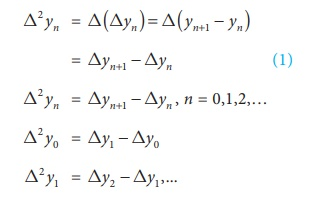Home | | Business Maths 12th Std | Forward Difference Operator(âˆ†)

# Forward Difference Operator(âˆ†)

The symbol Î” is called the forward difference operator and pronounced as delta.

Forward Difference Operator(âˆ† ):

Let y = f(x) be a given function of x. Let y 0 , y1 , y 2 , â€¦.. , yn be the values of y at = x 0 , x1 , x 2 ,â€¢, xn respectively. Then y1 âˆ’ y 0 , y 2 âˆ’ y1, y 3 âˆ’ y 2 , â€¦, y n âˆ’ ynâˆ’1 are called the first (forward) differences of the function y. They are denoted by Î”y 0 , Î”y1 , Î”y 2 ,..., Î”ynâˆ’1 respectively.

(i.e) Î”y 0 = y1 âˆ’ y 0 , Î”y1 = y 2 âˆ’ y1, Î”y 2 = y3 âˆ’ y 2 ,..., Î”yn âˆ’1 = y n âˆ’ ynâˆ’1

In general, Î”y n = yn+1 âˆ’ y n , n = 0,1,2,3,...

The symbol Î” is called the forward difference operator and pronounced as delta.

The forward difference operator âˆ† can also be defined as Df ( x) = f ( x + h ) âˆ’ f ( x), h is the equal interval of spacing.

## Proof of these properties are not included in our syllabus:

Properties of the operator Î” :

Property 1: If c is a constant then Î”c = 0

Proof: Let f (x) = c

âˆ´ f ( x + h ) = c (where â€˜hâ€™ is the interval of difference)

Î”f ( x) = f ( x + h ) âˆ’ f ( x)

Î”c = c âˆ’ c = 0

Property 2: Î” is distributive i.e. Î” ( f (x) + g (x)) = Î” f (x) + Î” g (x)

Proof: Î”[f (x) + g (x)] = [f (x + h ) + g(x + h) ] â€“ [f (x)+ g (x)]

= f (x + h ) + g(x + h) âˆ’ f (x) âˆ’ g (x)

= f (x + h ) âˆ’ f (x )+ g(x + h) âˆ’ g (x)

= Î” f (x) + Î” g (x)

Similarly we can show that Î” [f ( x) âˆ’ g ( x)] = f (x) âˆ’ Î” [g ( x)]

In general, Î”[ f1 (x) + f 2 (x)...... + f n (x) ] = Î” f1 (x)+ Î” f 2 (x) + .... + Î” f n (x)

Property 3: If c is a constant then Î” c f ( x ) = c Î” f ( x)

Proof: Î” [c f (x)] = c f ( x + h) âˆ’ c f ( x)

= c [ f (x + h) âˆ’ f (x)]

= c Î” f (x)

## Results without proof

1.        If m and n are positive integers then Î” m . Î” n f (x) = Î” m +n f (x)

2.        Î”[ f (x) g (x) ] = f (x) g (x) + g (x) Î” f (x)

3.The differences of the first differences denoted by Î”2y0, Î”2y1, â€¦., Î”2yn, are called second differences, whereSimilarly the differences of second differences are called third differences.It is convenient to represent the above differences in a table as shown below.

## Forward Difference Table for y :## The forward difference table for f(x) is given below.Tags : Finite Differences | Numerical Methods , 12th Business Maths and Statistics : Chapter 5 : Numerical Methods
Study Material, Lecturing Notes, Assignment, Reference, Wiki description explanation, brief detail
12th Business Maths and Statistics : Chapter 5 : Numerical Methods : Forward Difference Operator(âˆ†) | Finite Differences | Numerical Methods